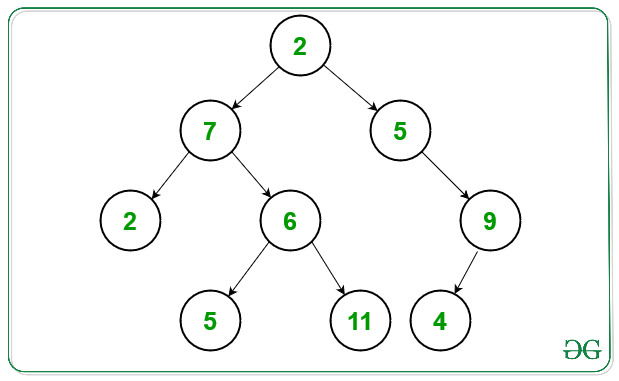Open in App
Not now

# Bottom-left to upward-right Traversal in a Binary Tree

• Difficulty Level : Expert
• Last Updated : 10 Jun, 2022

Given a Binary Tree, the task is to print the Bottom-left to Upward-right Traversal of the given Binary Tree i.e., the level order traversal having level as Bottom-left to Upward-right node.

Examples:

Input: Below is the given Tree:Output: 2 7 2 5 6 5 11 4 9
Explanation:

Level 1: 2 7 2 (going upwards from bottom left to right to root)
Level 2: 5 6 5 (right from each node in layer 1/or bottom left to upwards right in this layer)
Level 3: 11 4 9 (right from each node in layer 2/or bottom left to upwards right in this layer)

Input: 1 2 3 4 5 6 7
Output: 4 2 1 5 6 3 2
Explanation
Layer 1: 4 2 1 (going upwards from bottom left to right to root)
Layer 2: 5 6 3 (right from each node in layer 1/or bottom left to upwards right in this layer)
Layer 3: 2 (right from each node in layer 2/or bottom left to upwards right in this layer)

Approach: The idea is to use the Breadth-First Search technique. Follow the steps needed to solve this problem:

• Initialize a layer in a binary tree. It is a list of nodes starting from the bottom-left most node next to the previous layer and ends with the upper-right most node next to the previous layer.
• Create a stack to stores all nodes in every layer.
• Initialize a queue to maintain “roots” in each layer, a root in a layer is a node from which one may go downwards using left children only.
• Push the root node of the first layer (the tree root) in the queue.
• Define an indicator (say lyr_root) a node expected at the end of a layer which is the current layer head, a layer head is the first node in a layer.
• Traverse until the queue is nonempty and do the following:
• Get a layer root from the front of the queue
• If this layer root is the layer head of a new layer, then, pop every element in the stack i.e., of the previous layer element, and print it.
• Traverse the layer from the upper-right to the bottom-left and for each element, if it has a right child, then check if the traversed node is the layer head or not. If found to be true then, change the expected indicator to indicate to the next layer head.
• Push the right child to the root in the queue.
• Push the traversed node in the stack.
• After traversing all the layers, the final layer may still be in the stack, so we need to pop every element from it and print it.

Below is the implementation of the above approach:

## C++

 `// C++ program for the above approach``#include ``#include ``using` `namespace` `std;` `// Node Structures``typedef` `struct` `Node {``    ``int` `data;``    ``Node* left;``    ``Node* right;``} Node;` `// Function to add the new Node in``// the Binary Tree``Node* newNode(``int` `data)``{``    ``Node* n;` `    ``// Create a new Node``    ``n = ``new` `Node();``    ``n->data = data;``    ``n->right = NULL;``    ``n->left = NULL;``    ``return` `n;``}` `// Function to traverse the tree in the``// order of bottom left to the upward``// right order``vector<``int``>``leftBottomTopRightTraversal(Node* root)``{``    ``// Stores the data of the node``    ``vector<``int``> rr;` `    ``// Stores every element in each layer``    ``stack<``int``> r;` `    ``// Stores the roots in the layers``    ``queue roots;` `    ``// Push the layer head of the``    ``// first layer``    ``roots.push(root);` `    ``// Define the first layer head``    ``// as the tree root``    ``Node* lyr_root = root;` `    ``// Traverse all layers``    ``while` `(!roots.empty()) {` `        ``// get current layer root``        ``Node* n = roots.front();` `        ``// Pop element from roots``        ``roots.pop();``        ``if` `(lyr_root == n) {` `            ``// Layer root was also``            ``// the layer head``            ``while` `(!r.empty()) {` `                ``rr.push_back(r.top());` `                ``// Pop every element``                ``// from the stack``                ``r.pop();``            ``}``        ``}` `        ``while` `(n) {` `            ``if` `(n->right) {` `                ``// Current traversed node``                ``// has right child then``                ``// this root is next layer``                ``if` `(n == lyr_root) {``                    ``lyr_root = n->right;``                ``}` `                ``// Push the right child``                ``// to layer roots queue``                ``roots.push(n->right);``            ``}` `            ``// Push node to the``            ``// layer stack``            ``r.push(n->data);``            ``n = n->left;``        ``}``    ``}` `    ``// Insert all remaining elements``    ``// for the traversal``    ``while` `(!r.empty()) {` `        ``// After all of the layer``        ``// roots traversed check the``        ``// final layer in stack``        ``rr.push_back(r.top());``        ``r.pop();``    ``}` `    ``// Return the traversal of nodes``    ``return` `rr;``}` `// Function that builds the binary tree``// from the given string``Node* buildBinaryTree(``char``* t)``{``    ``Node* root = NULL;` `    ``// Using queue to build tree``    ``queue q;``    ``int` `data = 0;` `    ``// Stores the status of last``    ``// node to be ignored or not``    ``bool` `ignore_last = ``false``;``    ``while` `(*t != ``'\0'``) {``        ``int` `d = *t - ``'0'``;` `        ``// If the current character``        ``// is a digits then form the``        ``// number of it``        ``if` `(d >= 0 && d <= 9) {``            ``data *= 10;``            ``data += d;``            ``ignore_last = ``false``;``        ``}` `        ``// If the current character``        ``// is N then it is the``        ``// NULL node``        ``else` `if` `(*t == ``'N'``) {``            ``data = 0;``            ``q.pop();``            ``ignore_last = ``true``;``        ``}` `        ``// If space occurred then``        ``// add the number formed``        ``else` `if` `(*t == ``' '``) {` `            ``// If last is ignored``            ``if` `(!ignore_last) {` `                ``// If root node is not NULL``                ``if` `(root) {` `                    ``Node** p = q.front();``                    ``q.pop();` `                    ``if` `(p != NULL) {``                        ``*p = newNode(data);``                        ``q.push(&((*p)->left));``                        ``q.push(&((*p)->right));``                    ``}``                ``}` `                ``// Else create a new``                ``// root node``                ``else` `{``                    ``root = newNode(data);``                    ``q.push(&(root->left));``                    ``q.push(&(root->right));``                ``}``                ``data = 0;``            ``}``        ``}` `        ``// Increment t``        ``t++;``    ``}` `    ``// Return the root node of the tree``    ``return` `root;``}` `// Driver Code``int` `main()``{``    ``// Given order of nodes``    ``char` `T[] = ``"2 7 5 2 6 N 9 N N 5 11 4 N"``;` `    ``// Builds the Binary Tree``    ``Node* root = buildBinaryTree(T);` `    ``// Function Call``    ``vector<``int``> result``        ``= leftBottomTopRightTraversal(root);` `    ``// Print the final traversal``    ``for` `(``int` `i = 0; i < result.size(); ++i) {``        ``cout << result[i] << ``" "``;``    ``}` `    ``return` `0;``}`

Output:

`2 7 2 5 6 5 11 4 9`

Time Complexity: O(N)
Auxiliary Space: O(N)

My Personal Notes arrow_drop_up